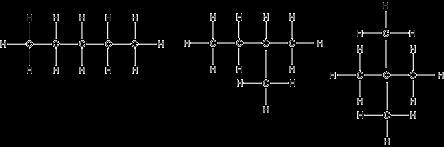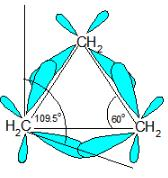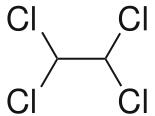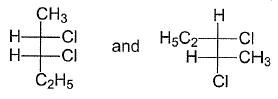Courses

# Test: Conformational Analysis- 1

## 10 Questions MCQ Test Chemistry for JEE | Test: Conformational Analysis- 1

Description
This mock test of Test: Conformational Analysis- 1 for JEE helps you for every JEE entrance exam. This contains 10 Multiple Choice Questions for JEE Test: Conformational Analysis- 1 (mcq) to study with solutions a complete question bank. The solved questions answers in this Test: Conformational Analysis- 1 quiz give you a good mix of easy questions and tough questions. JEE students definitely take this Test: Conformational Analysis- 1 exercise for a better result in the exam. You can find other Test: Conformational Analysis- 1 extra questions, long questions & short questions for JEE on EduRev as well by searching above.
QUESTION: 1

### Direction (Q. Nos. 1-10) This section contains 10 multiple choice questions. Each question has four  choices (a), (b), (c) and (d), out of which ONLY ONE option is correct. The molecular formula C5H12 contains how many isomeric alkanes?

Solution:

The correct answer is Option C.
From the image we can see that 3 isomers are possible for pentane.QUESTION: 2

Solution:
QUESTION: 3

### Which of the following cycloalkanes exhibits the greatest molar heat of combustion per —CH2 — group?

Solution:

The correct answer is option D
Cyclopropane is a cycloalkane molecule with the molecular formula C3H6, consisting of three carbon atoms linked to each other to form a ring, with each carbon atom bearing two hydrogen atoms resulting in D3H molecular symmetry. The small size of the ring creates substantial ring strain in the structure.

QUESTION: 4

Which of the following correctly ranks the cycloalkanes in order of increasing ring strain per methylene group?

Solution:

The correct answer is Option B.The C-C-C bond angles in cyclopropane (60o) and cyclobutane (90o) are much different than the ideal bond angle of 109.5o.This bond angle causes cyclopropane and cyclobutane to have a high ring strain. However, molecules, such as cyclohexane and cyclopentane, would have a much lower ring strain because the bond angle between the carbons is much closer to 109.5o.

QUESTION: 5

Which,of the following correctly lists the conformations of cyclohexane in order of increasing potential energies?

Solution:

Chair >Twist boat > Boat > Half-chair

above is the stability order of
the conformed.
Stability is inversely proportional to potential energy.

QUESTION: 6

Which is not true regarding conformers of ethane?

Solution:

The correct answer is Option D.
Although conformers differ in potential energy and stability, the difference is so small that it does not allow their practical separation.

QUESTION: 7

The correct statement concerning conformers of 1,2-dichloroethane is

Solution:
QUESTION: 8

Which of the following molecule exhibits conformational isomerism ?

Solution:

The correct answer is option A
Conformational isomers. Methane (CH4) is a molecule that is a perfect tetrahedron, and so it is commonly said that no isomerism is possible with methane. However, the carbon-hydrogen bonds of methane constantly vibrate and bend, so that on very short timescales an apparent isomerism can be said to exist.

QUESTION: 9

Which is true about conformers of 1,1,2,2-tetrachloroethane?

Which is true about conformers of 1,1,2,2-tetrachloroethane?
a) The most stable conformer has dihedral angle of 60° between all adjacent chlorine atoms
b) In the least stable conformer, two Cl-atoms are eclipsing one another while other two Cl-atoms are eclipsed to hydrogen atoms
c) In the most stable conformer, dihedral angle between hydrogen atoms is 60°
d) The most stable conformer is non-polar

Solution:

The correct answer is option DThis molecule is non-polar two Cl atoms in one carbon atom cancel the polarity other two Cl atoms on the next carbon.
Also all the Cl atoms are at max distance from each other so max. Stability due to less repulsion between Cl atoms. Dihedral angle between H and Cl is 60°

QUESTION: 10

What is relationship between the following Fischer Projections?Solution:

The correct answer is option C
Conformers are isomers having the same bond connectivity sequence and can be interconverted by rotation around one or more single (σ) bonds. Hence, the two structures represent conformers.